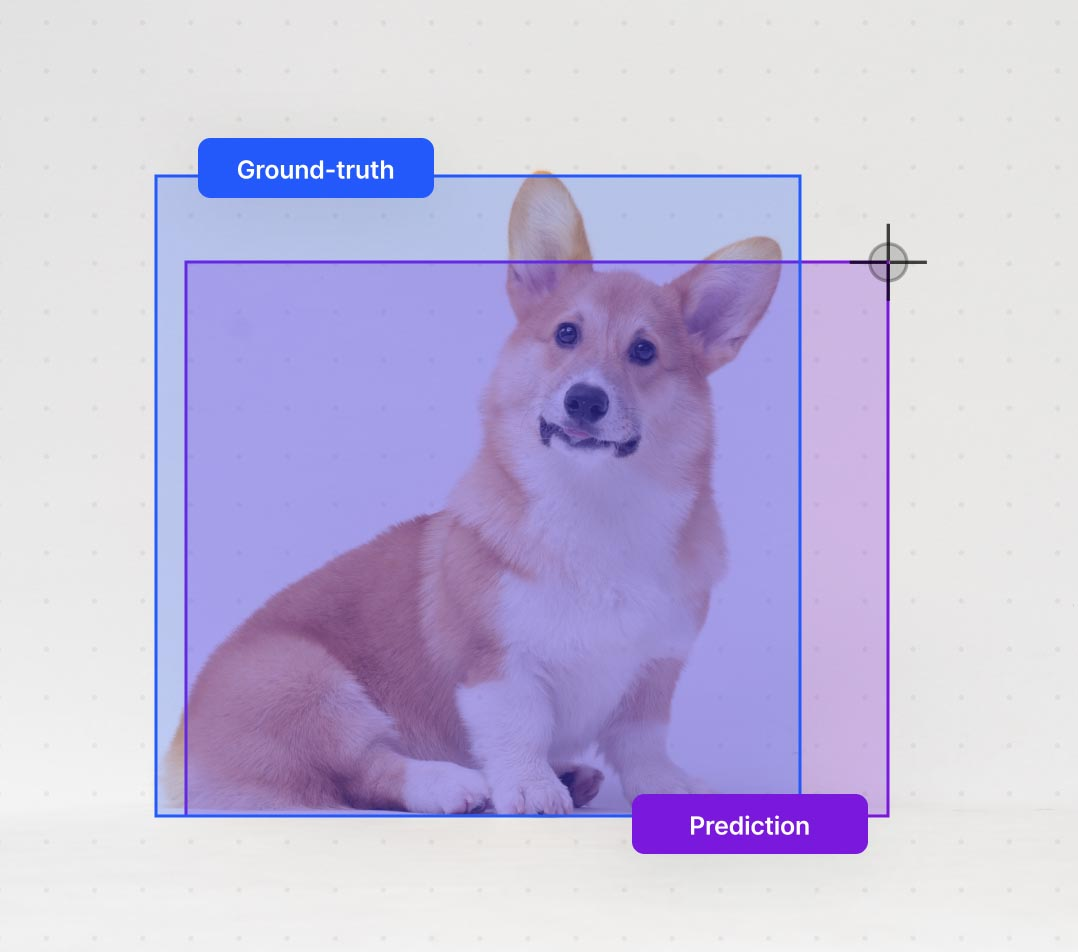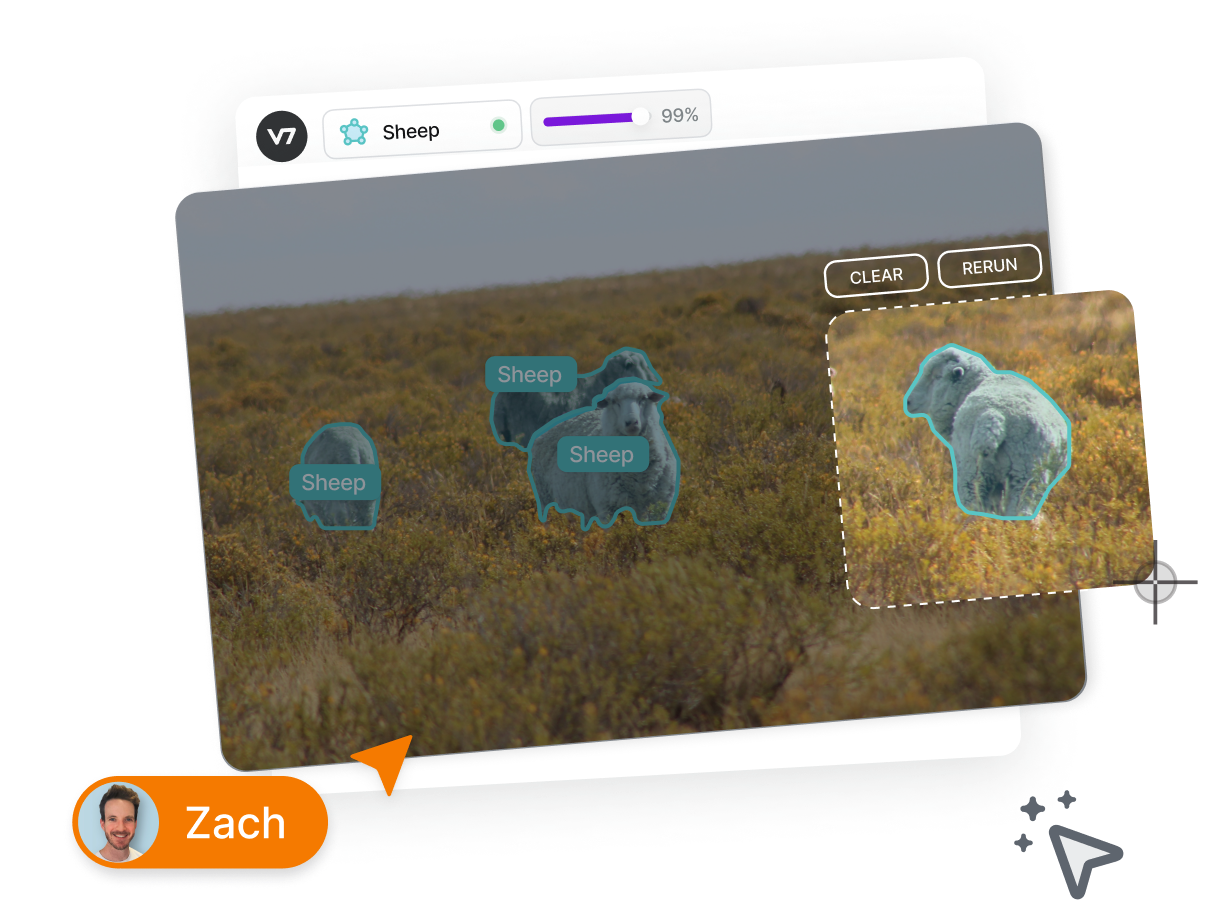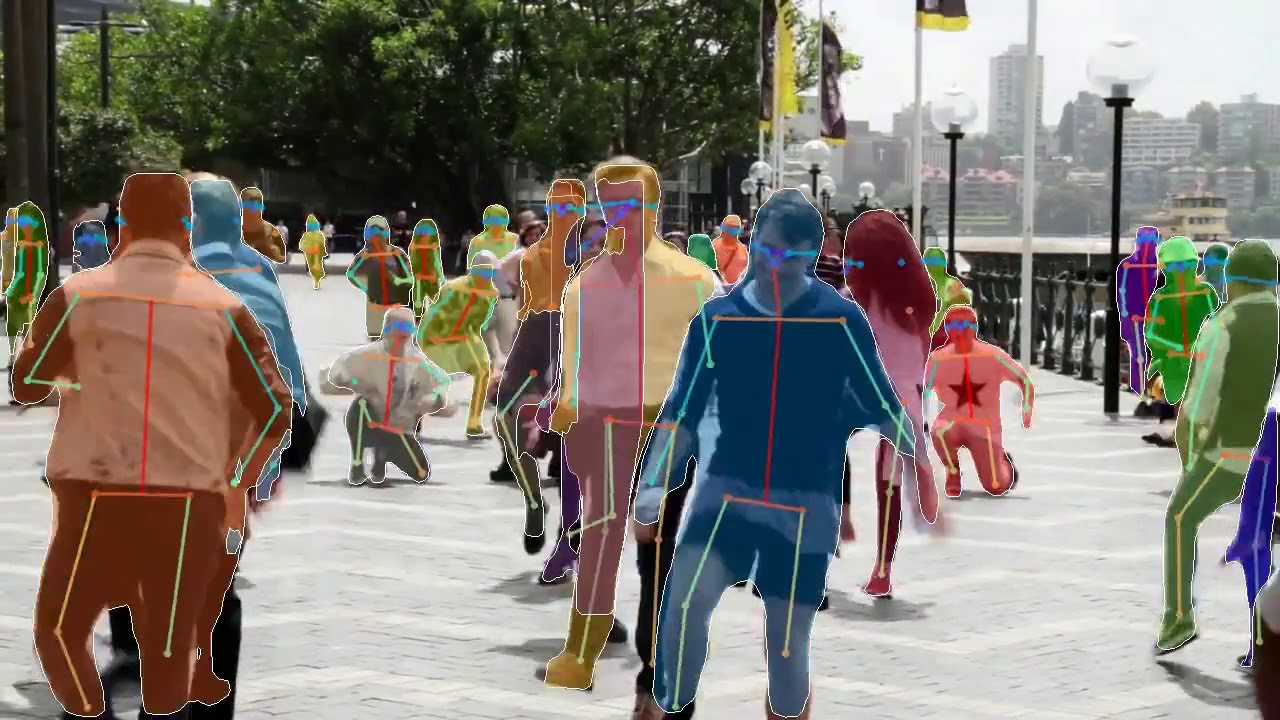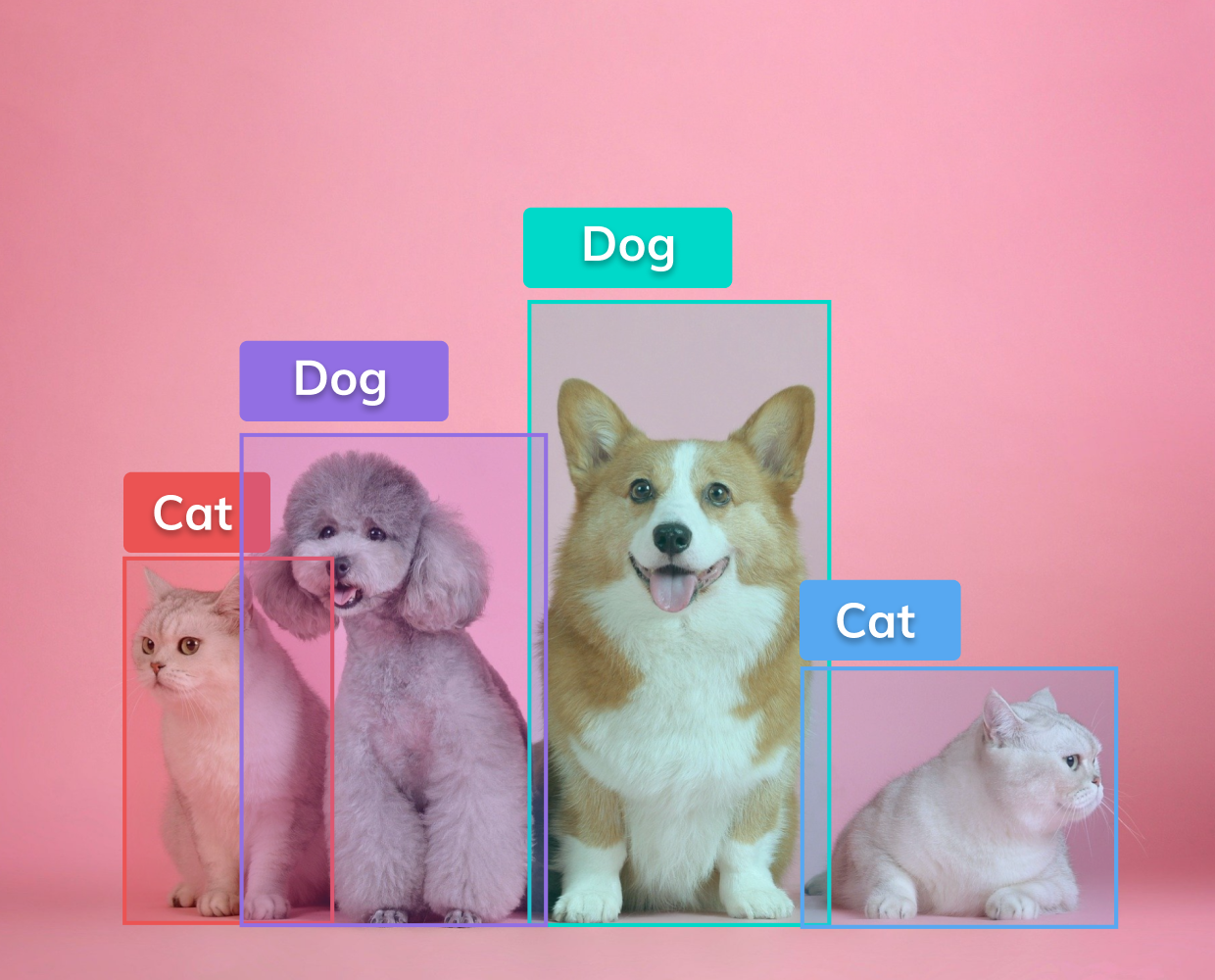# Intersection over Union (IoU): Definition, Calculation, Code

Find out how and when to use IoU, one of the most crucial model model assessment metrics.May 30, 2023Identifying and localizing things inside an image or video is a core computer vision task. Among these tasks, one of the most popular ones is object detection.

Like every machine learning model, object detection models require a set of metrics to assess their accuracy. AP (Average Precision) and IoU (Intersection over Union) are commonly used metrics. In this article, we’ll dive deeper into the latter.

IoU is a crucial metric for assessing segmentation models, commonly called Jaccard's Index, since it quantifies how well the model can distinguish objects from their backgrounds in an image. It’s used in numerous computer vision applications, such as autonomous vehicles, security systems, and medical imaging.

Here’s what we’ll cover:

Let’s go!

Train ML models and solve any computer vision task faster with V7.

Don't start empty-handed. Explore our repository of 500+ open datasets and test-drive V7's tools.

## What is Intersection over Union?

Intersection over Union is a popular metric to measure localization accuracy and compute localization errors in object detection models. It calculates the amount of overlapping between two bounding boxes—a predicted bounding box and a ground truth bounding box.

IoU is the ratio of the intersection of the two boxes' areas to their combined areas. The ground truth bounding box and the anticipated bounding box both encompass the area of union, which is the denominator.

We calculate the overlap between the ground-truth bounding box and the predicted bounding box in the numerator. Mathematically, it is written as:

But for binary classification, it is written as:

Where

• TP= True Positive.
• FN= False Negative
• FP= False Positive

Here’s the visual representation of IoU:

The IoU score will be high if there is much overlap between the anticipated and ground truth boxes. In contrast, a low overlap will result in a low IoU score. An IoU score of 1 indicates a perfect match between the projected box and the ground truth box, whereas a score of 0 means no overlapping between the boxes.

Let's look at a straightforward object detection example to understand it better.

Imagine you wanted to use a deep learning model to identify a sleeping dog in the image below. The model will produce an estimated bounding box for the dog. However, the real ground truth box that has been carefully annotated from around the dog may not exactly match this forecast box. To assess the model's accuracy, the IoU measure determines how much the forecasted box coincides with the actual box.

In the figure above, three instances are seen to be emerged after calculating the IoU. In the first instance of the dog sleeping, the model works almost perfectly, indicating a greater accuracy. The second instance, with an IoU of 0.79, is average. Finally, in the third instance, it performs poorly with an IoU of 0.45, showing that the object is not detected properly.

The IoU metric is helpful since it offers a numerical assessment of how well a model identifies items in an image.

Additionally, while training your model, you can choose a minimum IoU score needed for a predicted box to be regarded as an accurate positive detection, which allows using IoU to set a threshold for object detection. You can manage the trade-off between detection accuracy and false positives by choosing the suitable threshold.

There is no one-size-fits-all recommended threshold for IoU, as it largely depends on the specific object detection task and dataset. However, a common threshold used in practice is 0.5, meaning that a predicted box must have an IoU of at least 0.5 with a ground truth box to be considered a true positive detection.

This threshold can be adjusted based on the desired trade-off between precision and recall. For example, increasing the threshold would result in fewer false positives but may also miss some true positives. It's essential to evaluate your model's performance on a validation set using different IoU thresholds to choose the most appropriate one for your task.

Keeping that in mind, IoU is an essential statistic for object detection and other computer vision applications in general. It enables us to assess the effectiveness of our algorithms and establish suitable detection accuracy standards.

## How to calculate IoU?

IoU is determined by calculating the overlap among two bounding boxes, a predicted box and a ground truth box.

Let's look at a mathematical derivation to understand IoU.

With the provided boxes X and Y, where,

$X=\left({A}_{1},{B}_{1},{C}_{1},{D}_{1}\right)$

$Y=\left({A}_{2},{B}_{2},{C}_{2},{D}_{2}\right)$

${\mathrm{A}}_{\mathrm{inter}}$ and ${\mathrm{B}}_{\mathrm{inter}}$ stand for the bounding box's top-left corner coordinates, while ${\mathrm{C}}_{\mathrm{inter}}$ and ${\mathrm{D}}_{\mathrm{inter}}$ stand for the bounding box's bottom-right corner coordinates.

${A}_{inter}=max\left({A}_{1},{A}_{2}\right)$

${B}_{inter}=max\left({B}_{1},{B}_{2}\right)$

${C}_{inter}=max\left({C}_{1},{C}_{2}\right)$

${D}_{inter}=max\left({D}_{1},{D}_{2}\right)$

There is one condition that needs to be met: which is that if the value of either $\left({\mathrm{C}}_{\mathrm{inter}}<{\mathrm{A}}_{\mathrm{inter}}\right)$ or $\left({\mathrm{D}}_{\mathrm{inter}}<{\mathrm{B}}_{\mathrm{inter}}\right)$ is less than zero, the intersection between the two boxes is zero, and the IoU score will be zero.

When the boxes don’t intersect, IoU cannot be calculated. If they do, the process of computing IoU is continued.

The overlap and the union parts of boxes X and Y are calculated. Equation (10) help us calculate the intersection points using the overlap boundary points, whereas equations (11) and (12) are used for calculating n(X) and n(Y) of the set X and Y.

Equations 13, on the other hand, is the union formula which is the sum of individual n(X) and n(Y) minus the intersection of both. Using Eq. (12) and Eq. (10), we can calculate Eq. (14).

Let's say that the ground truth bounding box for a picture of a dog is [A1=50, B1=100, C1=200, D1=300] and the predicted bounding box is [A2=80, B2=120, C2=220, D2=310].

The visual representation of the box is shown below:

Before the area of union is computed, you must first find the smallest bounding box that contains both the expected and ground truth bounding boxes.

${A}_{inter}=\left(50,80\right)=80$

${B}_{inter}=\left(100,120\right)=120$

${C}_{inter}=min\left(200,220\right)=200$

${D}_{inter}=\left(300,310\right)=300$

As value 200 > 80 and 300 > 120 proves that the boxes intersect, IoU can be calculated. The calculation is as follows:

To calculate the IoU score, we can now enter the following values into the IoU formula:

As a result, the IoU score for this instance is 0.62, suggesting barely any overlap between the anticipated and actual bounding boxes.

Here is the pseudo-code for calculating IoU:

function compute_iou(box1, box2):
# Calculate intersection area
intersection_width = min(box1.right, box2.right) - max(box1.left, box2.left)
intersection_height = min(box1.bottom, box2.bottom) - max(box1.top, box2.top)

if intersection_width <= 0 or intersection_height <= 0:
return 0

intersection_area = intersection_width * intersection_height

# Calculate union area
box1_area = (box1.right - box1.left) * (box1.bottom - box1.top)
box2_area = (box2.right - box2.left) * (box2.bottom - box2.top)

union_area = box1_area + box2_area - intersection_area

# Calculate IoU
iou = intersection_area / union_area
return iou


## Where to get ground truth data from?

Ground-truth data in Intersection over Union (IoU) refers to the actual or accurate values of the evaluated objects or areas. The ground-truth data compares the anticipated values produced by a modeling or algorithm.

In object detection, for example, the ground-truth information would be the precise bounding boxes surrounding the items of interest in an image. Human experts manually mark or label these boundary boxes. The IoU score is calculated by comparing the predicted bounding boxes produced by an object detection model to the ground-truth bounding boxes during evaluation.

In other tasks, such as semantic or instance segmentation, the ground-truth data consists of the true class labels and segmentations of pixels or regions in a picture. The IoU score is then computed by contrasting the anticipated and ground-truth segmentations.

It is critical to have precise and trustworthy ground-truth data to assess the efficiency of machine learning algorithms and models and compare several models or algorithms to discover which works best.

The ground truth dataset for calculating IoU may vary depending on the task. For example, in object detection, the ground truth dataset would consist of precise bounding boxes manually marked by human experts around the objects of interest in an image. In contrast, in semantic or instance segmentation, the ground truth dataset would comprise the true class labels and segmentations of pixels or regions in an image. Therefore, preparing ground truth data will depend on the specific task and the data type being evaluated.

1. Collect the dataset: First, you must gather the photos containing the items that need to be detected. You can use publicly accessible datasets or build their dataset by collecting and classifying images.

##### 💡 Pro tip: Check out of V7’s 500+ open datasets

2. Gather the data and add annotations: The objects in the photographs might need to be labeled and their locations marked with bounding boxes. This can be done manually or with the help of an annotation tool—such as V7.

##### 💡 Pro tip: Check out our image annotation guide & video annotation guide to see how to label your data effectively.
Label 10x faster with auto-annotate

Pixel-perfect annotation quality, increased efficiency, and lowered overheads.3. Take note of the bounding box coordinates: Take note of the bounding box coordinates for every object in each image. In most cases, the coordinates are written as (x, y, width, height), where (x, y) stands for the coordinates of the bounding box's top-left corner and (width, height) for the bounding box's width and height.

4. Keep the actual data at hand: In a structured file format, such as a CSV or JSON file, save all the locations of the object inside the image, together with its matching bounding box coordinates, along with the object class or category information. You can use it later to evaluate the model's accuracy for each call or category.

5. Split the dataset into training and testing sets: Create training and test sets from the dataset. The object detection model is trained on the training set, and its performance is assessed on the test set using the IoU metric.

##### 💡 Pro tip: Most model training tools, such as V7, automatically split data into validation, train, and test sets.

6. Prepare the prediction data: If a model can forecast the object's location, it must save its findings in a format corresponding to the ground truth data.

7. Calculate IoU score: Compute the IoU score for each object in the dataset once we possess the ground truth and predicted bounding boxes. The intersection area of the two bounding boxes is divided by the area of its union to determine the IoU score.

8. Evaluate the findings: The IoU ratings can be used to assess the model's accuracy and, if necessary, suggest changes.

## Key takeaways

• Intersection over Union (IoU) is a widely-used evaluation metric in object detection and image segmentation tasks.
• IoU measures the overlap between predicted bounding boxes and ground truth boxes, with scores ranging from 0 to 1.
• The IoU metric is essential for comparing and evaluating the performance of detection models.
• To compute IoU, calculate the intersection and union areas between the predicted and ground truth bounding boxes.
• Preparing a labeled dataset with training, testing, and optional validation sets is necessary for training an object detection model.Deval is a senior software engineer at Eagle Eye Networks and a computer vision enthusiast. He writes about complex topics related to machine learning and deep learning.

“Collecting user feedback and using human-in-the-loop methods for quality control are crucial for improving Al models over time and ensuring their reliability and safety. Capturing data on the inputs, outputs, user actions, and corrections can help filter and refine the dataset for fine-tuning and developing secure ML solutions.”Name
Company
GUIDE
Building AI-Powered Products: The Enterprise Guide

Building AI products? This guide breaks down the A to Z of delivering an AI success story.

Oops! Something went wrong while submitting the form.## Related articlesA Comprehensive Guide to Human Pose Estimation
Nilesh Barla10The Ultimate Guide to Object Detection
Alberto Rizzoli9
Gain control of your training data
15,000+ ML engineers can’t be wrongGUIDE
FREE
Building AI-Powered Products: The Enterprise Guide
A Comprehensive Guide

Building AI products? This guide breaks down the A to Z of delivering an AI success story.